# De Morgan's Theorems

De Morgan has suggested two theorems which are extremely useful in Boolean Algebra. The two theorems are discussed below.

## Theorem 1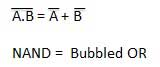• The left hand side (LHS) of this theorem represents a NAND gate with inputs A and B, whereas the right hand side (RHS) of the theorem represents an OR gate with inverted inputs.

• This OR gate is called as Bubbled OR.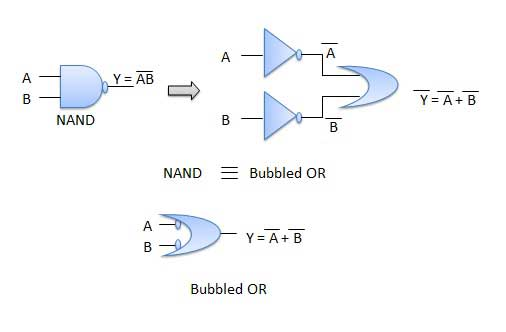Table showing verification of the De Morgan's first theorem −## Theorem 2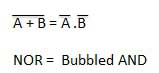• The LHS of this theorem represents a NOR gate with inputs A and B, whereas the RHS represents an AND gate with inverted inputs.

• This AND gate is called as Bubbled AND.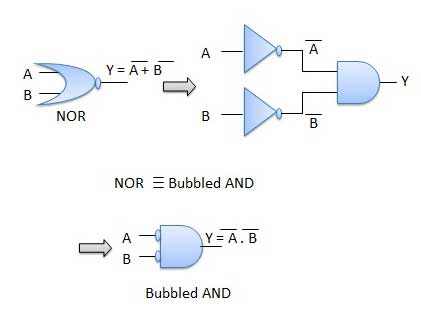Table showing verification of the De Morgan's second theorem −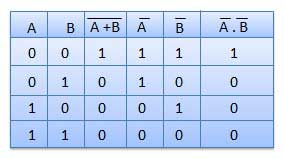boolean_algebra.htm